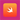# Swift Switch-Case문

• 스위프트에도 여느 프로그래밍 언어와 마찬가지로 조건문이 있다.
• 대표적으로 if-else문과 switch-case문이 사용된다.
• if-else문은 지난 글을 통해 알아봤다.
• 이번에는 switch-case문에 대해 알아보겠다.

## Switch문

• 패턴 비교문이라고도 하는 Switch문은 가장 첫 번째 매칭되는 패턴의 구문이 실행된다.
• Switch문의 문법과 예제를 통해 조금 더 살펴보도록 하자.

## Switch문 문법

``````switch value
{
case value1:
respond to value 1
case value2,
value3:
respond to value 2 or 3
default:
otherwise, do something else
}``````
• 각 상태는 키워드 case를 통해 나타낼수 있다.
• case 상태 끝에 콜론 ':'을 붙여 패턴을 종료한다.
• 하나의 case문이 종료되면 switch문이 종료된다.
• 또한 switch문은 모든 경우를 커버하기 위해서 마지막에 default 키워드를 사용해야 한다.
• 단, default 키워드 없이도 모든 경우가 커버 되었다면, default 키워드가 없어도 된다.

## Switch문 Interval Matching

• Switch문 Interval Matching이란 범위 연산자를 활용해,
• 단순히 값을 매칭하는 것을 넘어 다양한 패턴을 통해 매칭하는 것을 말한다.
``````func interSwitch(count:Int)
{
let countedThings = "moons orbiting Saturn"
let naturalCount: String

switch count
{
case 0:
naturalCount = "no"
case 1..<5:

naturalCount = "a few"
case 5..<12:

naturalCount = "several"
case 12..<100:

naturalCount = "dozens of"
case 100..<1000:

naturalCount = "hundreds of"
default:
naturalCount = "many"
}

print("There are \(naturalCount) \(countedThings).")
}``````

## Switch문 튜플 매칭

• 튜플과 와일드카드를 이용해서 Switch문에서 여러개의 값을 동시에 확인할 수 있다.
``````func getPoint(somePoint:(Int,Int))
{
switch somePoint
{
case (0, 0):
print("\(somePoint) is at the origin")
case (_, 0):
print("\(somePoint) is on the x-axis")
case (0, _):
print("\(somePoint) is on the y-axis")
case (-2...2, -2...2):
print("\(somePoint) is inside the box")
default:
print("\(somePoint) is outside of the box")
}
}``````

## Switch문 값 바인딩

• Switch문에서 값 바인딩을 통해 case 내부에서 사용되는 임시 값으로 매칭 시킬 수 있다.
``````func getPoint(somePoint:(Int,Int))
{
switch somePoint
{
case (0, 0):
print("\(somePoint) is at the origin")
case (let x, 0):
print("on the x-axis with an x value of \(x)")
case (0, let y):
print("on the y-axis with an y value of \(y)")
case (-2...2, -2...2):
print("\(somePoint) is inside the box")
default:
print("\(somePoint) is outside of the box")
}
}``````

## Switch문 where문

• where문의 추가로 조건을 더욱 세부화할 수도 있다.
``````func wherePoint(point:(Int,Int))
{
switch point
{
case let (x, y) where x == y:
print("(\(x), \(y)) is on the line x == y")
case let (x, y) where x == -y:
print("(\(x), \(y)) is on the line x == -y")
case let (x, y):
print("(\(x), \(y)) is just some arbitrary point")
}
}``````